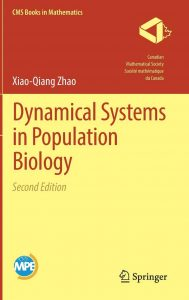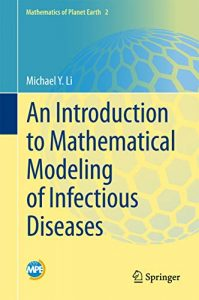This column features three recent books related to a very topical subject, written (or co-authored) by Canadian researchers. Xiao-Qiang Zhao is Professor of Mathematics at Memorial University; his book, now in its second edition, was recently reviewed in the Notes. Fred Brauer is Honourary Professor in the Mathematical Biology group at UBC Vancouver. Michael Y. Li is Professor of Mathematics at the University of Alberta. Full reviews of the second and third of these books may be published in future issues of the Notes.

Karl Dilcher, Dalhousie University (notes-reviews@cms.math.ca)Dynamical Systems in Population Biology, 2nd Edition
by Xiao-Qiang Zhao
CMS Books in Mathematics, Springer, 2017
ISBN 978-3-319-56432-6
Reviewed by Karl Dilcher

A full review, written by Frithjof Lutscher, was recently published in the March/April 2020 issue of the Notes. The following paragraph is worth repeating here:

Chapter 11 is devoted to a quantity of great interest in epidemiology: the basic reproduction ratio. This quantity is abstractly defined as the number of secondary infections that a single infective organism in a completely susceptible population will generate. Defining this quantity in models of great complexity, i.e. models that include spatial structure, delays, and interacting populations, is highly nontrivial. Proving that this number has the same properties as in the simple ODE models for which it was originally introduced, namely that it is the threshold between disease extinction and persistence, is very hard. It requires the tools and techniques introduced in the first chapters and several additional ideas. Chapters 12–14 then consider more applications of this basic reproduction ratio to populations with periodic delays, with spatial structure, and for the complicated dynamics of Lyme disease.Mathematical Models in Epidemiology
By Fred Brauer, Carlos Castillo-Chavez, and Zhilan Feng.
With a foreword by Simon Levin.
Texts in Applied Mathematics, 69. Springer, New York, 2019.
ISBN: 978-1-4939-9826-5
Reviewed by Karl Dilcher

Publisher’s description:

The book is a comprehensive, self-contained introduction to the mathematical modeling and analysis of disease transmission models. It includes (i) an introduction to the main concepts of compartmental models including models with heterogeneous mixing of individuals and models for vector-transmitted diseases, (ii) a detailed analysis of models for important specific diseases, including tuberculosis, HIV/AIDS, influenza, Ebola virus disease, malaria, dengue fever and the Zika virus, (iii) an introduction to more advanced mathematical topics, including age structure, spatial structure, and mobility, and (iv) some challenges and opportunities for the future.

There are exercises of varying degrees of difficulty, and projects leading to new research directions. For the benefit of public health professionals whose contact with mathematics may not be recent, there is an appendix covering the necessary mathematical background. There are indications which sections require a strong mathematical background so that the book can be useful for both mathematical modelers and public health professionals.An Introduction to Mathematical Modeling of Infectious Diseases
By Michael Y. Li
Mathematics of Planet Earth Collection, Springer, 2018.
ISBN 978-3-319-72122-4

Publisher’s Description:

This text provides essential modeling skills and methodology for the study of infectious diseases through a one-semester modeling course or directed individual studies.  The book includes mathematical descriptions of epidemiological concepts, and uses classic epidemic models to introduce different mathematical methods in model analysis.  Matlab codes are also included for numerical implementations.

It is primarily written for upper undergraduate and beginning graduate students in mathematical sciences who have an interest in mathematical modeling of infectious diseases.  Although written in a rigorous mathematical manner, the style is not unfriendly to non-mathematicians.

The individual chapters are: “Important Concepts in Mathematical Modeling of Infectious Diseases”; “Five Classic Epidemic Models and Their Analysis”; “Basic Mathematical Tools and Techniques”; “Parameter Estimation and Nonlinear Least-Squares Methods”; and “Special Topics”.